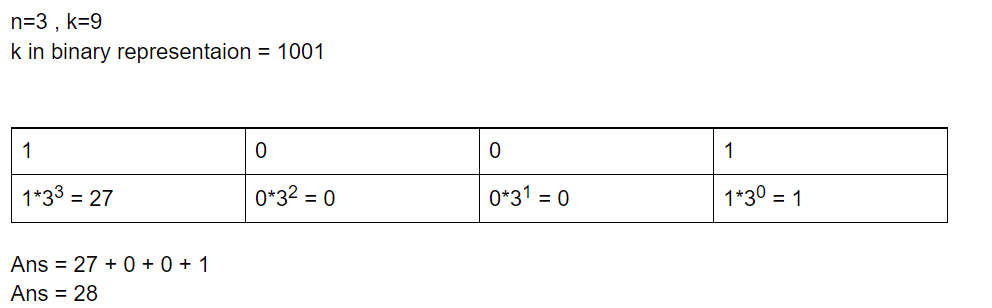# Find Kth number that can be written as sum of different powers of N

• Difficulty Level : Medium
• Last Updated : 13 Dec, 2021

Given two positive integers N and K. The task is to find the Kth number that can be written as the sum of different non-negative powers of N

Examples:

Input: N = 3, K = 4
Output: 9
Explanation:
First number that can be written as sum of powers of 3 is [1 = 30]
Second number that can be written as sum of powers of 3 is [3 = 31]
Third number that can be written as sum of powers of 3 is [4 = 30 + 31]
Fourth number that can be written as sum of powers of 3 is [9 = 32]

Input: N = 2, K = 12
Output: 12

Approach: This problem can be solved by using the concept of decimal to binary conversion.  The idea is to find the binary representation of K and start iterating from the least significant bit to the most significant bit. If the current bit is set then include the corresponding power of N to the answer otherwise skip that bit. Refer to the picture below for a better understanding.Example: When N = 3, K = 9

Below is the implementation of the above approach:

## C++

 `// C++ program for the above approach` `#include ` `using` `namespace` `std;`   `// Function to find Kth number that can be` `// represented as sum of different` `// non-negative powers of N` `int` `FindKthNum(``int` `n, ``int` `k)` `{` `    ``// To store the ans` `    ``int` `ans = 0;`   `    ``// Value of current power of N` `    ``int` `currPowOfN = 1;`   `    ``// Iterating through bits of K` `    ``// from LSB to MSB` `    ``while` `(k) {` `        ``// If the current bit is 1 then include` `        ``// corresponding power of n into ans` `        ``if` `(k & 1) {` `            ``ans = ans + currPowOfN;` `        ``}`   `        ``currPowOfN = currPowOfN * n;` `        ``k = k / 2;` `    ``}`   `    ``// Return the result` `    ``return` `ans;` `}`   `// Driver Code` `int` `main()` `{` `    ``int` `N = 3;` `    ``int` `K = 4;`   `    ``cout << FindKthNum(N, K);` `}`

## Java

 `// Java program for the above approach` `class` `GFG` `{` `  `  `  ``// Function to find Kth number that can be` `  ``// represented as sum of different` `  ``// non-negative powers of N` `  ``static` `int` `FindKthNum(``int` `n, ``int` `k)` `  ``{` `    `  `      ``// To store the ans` `      ``int` `ans = ``0``;`   `      ``// Value of current power of N` `      ``int` `currPowOfN = ``1``;`   `      ``// Iterating through bits of K` `      ``// from LSB to MSB` `      ``while` `(k > ``0``)` `      ``{` `        `  `          ``// If the current bit is 1 then include` `          ``// corresponding power of n into ans` `          ``if` `((k & ``1``) == ``1``) {` `              ``ans = ans + currPowOfN;` `          ``}`   `          ``currPowOfN = currPowOfN * n;` `          ``k = k / ``2``;` `      ``}`   `      ``// Return the result` `      ``return` `ans;` `  ``}` ` `  `  ``// Driver Code` `  ``public` `static` `void` `main(String []args)` `  ``{` `      ``int` `N = ``3``;` `      ``int` `K = ``4``;`   `      ``System.out.println(FindKthNum(N, K));` `  ``}`   `}`   `// This Code is contributed by ihritik`

## Python3

 `# Python program for the above approach`   `# Function to find Kth number that can be` `# represented as sum of different` `# non-negative powers of N` `def` `FindKthNum(n, k):`   `  ``# To store the ans` `  ``ans ``=` `0`   `  ``# value of current power of N` `  ``currPowOfN ``=` `1`   `  ``# Iterating through bits of K` `  ``# from LSB to MSB` `  ``while` `(k > ``0``):` `    `  `    ``# If the current bit is 1 then include` `    ``# corresponding power of n into ans` `    ``if` `((k & ``1``) ``=``=` `1``) :` `      ``ans ``=` `ans ``+` `currPowOfN` `    `    `    ``currPowOfN ``=` `currPowOfN ``*` `n` `    ``k ``=` `k ``/``/` `2` `  `    `  ``# Return the result` `  ``return` `ans`       `# Driver Code`   `N ``=` `3` `K ``=` `4` `print``(FindKthNum(N, K));` `  `    `# This Code is contributed by ihritik`

## C#

 `// C# program for the above approach` `using` `System;` `class` `GFG` `{` `  `  `  ``// Function to find Kth number that can be` `  ``// represented as sum of different` `  ``// non-negative powers of N` `  ``static` `int` `FindKthNum(``int` `n, ``int` `k)` `  ``{` `    `  `      ``// To store the ans` `      ``int` `ans = 0;`   `      ``// Value of current power of N` `      ``int` `currPowOfN = 1;`   `      ``// Iterating through bits of K` `      ``// from LSB to MSB` `      ``while` `(k > 0) ` `      ``{` `        `  `          ``// If the current bit is 1 then include` `          ``// corresponding power of n into ans` `          ``if` `((k & 1) == 1) {` `              ``ans = ans + currPowOfN;` `          ``}`   `          ``currPowOfN = currPowOfN * n;` `          ``k = k / 2;` `      ``}`   `      ``// Return the result` `      ``return` `ans;` `  ``}` ` `    `  ``// Driver Code` `  ``public` `static` `void` `Main()` `  ``{` `      ``int` `N = 3;` `      ``int` `K = 4;`   `      ``Console.WriteLine(FindKthNum(N, K));` `  ``}`   `}`   `// This Code is contributed by ihritik`

## Javascript

 ``

Output:

`9`

Time Complexity: O(log2K)

Space Complexity: O(1)

My Personal Notes arrow_drop_up
Recommended Articles
Page :